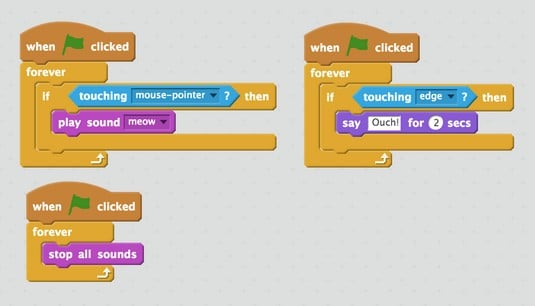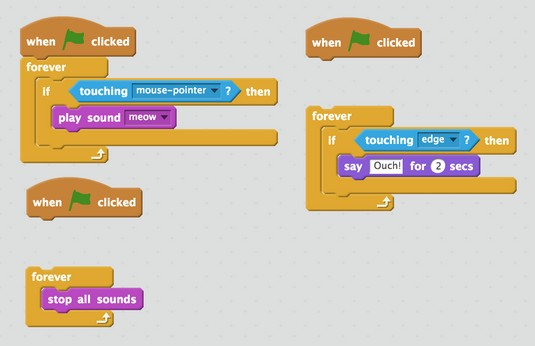##### Helping Kids with Coding For DummiesWhen you and your young coder are trying to debug, sometimes no error messages give you insight into the problem. Here, you find a list of strategies for debugging programs where you don’t get an error message, or the error message doesn’t give you enough information.

## Turning sections on and off

One of the best ways to debug is to disable sections of code so that you have small sections to test.

### Using Scratch

In Scratch, you might have a lot of scripts that start when the green flag is pressed. This can cause problems if some of the scripts cancel out or affect the other scripts. For example, the image below shows three scripts associated with one sprite.An example Scratch program.

The problem that the user notices is when the mouse pointer is touching the sprite, it does not meow. To try to figure out the problem, the coder might disconnect all the scripts and only have one script connected at a time. Then they see that the code for the mouse pointer touching the sprite works correctly, but that there is another problem (the code that stops all sounds forever). By connecting and disconnecting the blocks, the coder can identify the problem.An example of turning off sections in Scratch to identify a bug.

### Using App Inventor

Other block-based languages like App Inventor make turning sections on and off even easier! The image below shows how if you right-click a code block, you can disable that block. In this example, you might want to make sure the initial property settings work before creating the lists and hiding the items. Then, after you confirm the properties are set properly, you can enable the call to `setupLists` to make sure that works. Then you can enable the call to `hideItems` to make sure that works.

By turning off all sections and then turning each one on one at a time, it’s easier to find bugs.

### Using Python

Text-based languages have a similar way of turning sections on and off — you only have to comment out the lines of code. In Python, you can comment out a single line of code like this:
`#print 'Hi'`
And you can comment out multiple lines of code like this:
```"'
for x in range(0, 4):
print ('Hi ' + pets[x])
"'```
Commenting out code is how you “turn off” or “disable” parts of your code when you’re in a text-based language.

## Testing sample data

A common bug that coders run into is not testing data to make sure that the program works. This can especially be a problem if you’re writing programs that take user input. It’s important to make sure you and your young coder think about what kind of input you’re expecting, and test to make sure the input is handled correctly.

You might have a program that gets input from the user and prints what the user types, like this Python code:

```name = raw_input('What is your name? ‘)
print (‘Hi ' + name)```
It’s important to test to make sure that if you put the following types of input, they still do what you, as the coder, expect:
```Sarah
Sarah Guthals
13
11/15
Sarah 55 Guthals```
By mixing letters, spaces, numbers, and other symbols like / you’re ensuring that your program performs as expected. This type of testing is unit testing and ensures that small portions of your program execute correctly with varying input.

One of the most challenging aspects of coding is that the code is abstract and sometimes the data is hidden. This is especially tricky when you have complex data or are performing complex operations on data. By adding a number of output messages in your code, you can indicate when certain sections of code have been reached, or you can show the current values of certain variables at various points during execution.

An example of adding output messages to a program to gain insight in Python follows. Here, your goal is to write a program to solve an algebraic expression. For example:

```x = input('Provide a number for x: ')
y = input('Provide a number for y: ')
first = 2*x
second = 6*y
sum = first - second

print '2x + 6y = '
print (sum)```
There is an error in this program; instead of adding the first and second elements, the coder is accidentally subtracting. Though this error is fairly obvious because this example is small, it shows how a simple typo could completely change the output. If you run this code, you get results like:
```Provide a number for x: 2
Provide a number for y: 3
2x + 6y =
-14```
This is clearly wrong. 2*2 + 6*3 = 4 + 18 = 22, not -14. One way of debugging this code is to add output messages at each point. For example, you could change your code to:
```x = input('Provide a number for x: ')
print ('x: ')
print (x)y = input('Provide a number for y: ')
print ('y: ')
print (y)first = 2*x
print ('first: ')
print (first)second = 6*y
print ('second: ')
print (second)sum = first - secondprint "2x + 6y = "
print sum```
Then, when you run the code you get the following output:
```Provide a number for x: 2
x:
2
Provide a number for y: 3
y:
3
first:
4
second:
18
2x + 6y =
-14```
Then the coder can see that x, y, first, and second are all correct. This must mean that it’s just when the sum is calculated that there is an error.